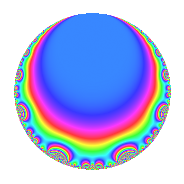# Properties

 Label 804.2.i.bLevel 804 Weight 2 Character orbit 804.i Analytic conductor 6.420 Analytic rank 0 Dimension 2 CM No Inner twists 2

# Related objects

## Newspace parameters

 Level: $$N$$ = $$804 = 2^{2} \cdot 3 \cdot 67$$ Weight: $$k$$ = $$2$$ Character orbit: $$[\chi]$$ = 804.i (of order $$3$$ and degree $$2$$)

## Newform invariants

 Self dual: No Analytic conductor: $$6.41997232251$$ Analytic rank: $$0$$ Dimension: $$2$$ Coefficient field: $$\Q(\sqrt{-3})$$ Coefficient ring: $$\Z[a_1, \ldots, a_{13}]$$ Coefficient ring index: $$1$$ Sato-Tate group: $\mathrm{SU}(2)[C_{3}]$

## $q$-expansion

Coefficients of the $$q$$-expansion are expressed in terms of a primitive root of unity $$\zeta_{6}$$. We also show the integral $$q$$-expansion of the trace form.

 $$f(q)$$ $$=$$ $$q - q^{3} + 2 q^{5} -5 \zeta_{6} q^{7} + q^{9} +O(q^{10})$$ $$q - q^{3} + 2 q^{5} -5 \zeta_{6} q^{7} + q^{9} -5 \zeta_{6} q^{11} + ( -3 + 3 \zeta_{6} ) q^{13} -2 q^{15} + ( -3 + 3 \zeta_{6} ) q^{17} + ( -7 + 7 \zeta_{6} ) q^{19} + 5 \zeta_{6} q^{21} + ( -3 + 3 \zeta_{6} ) q^{23} - q^{25} - q^{27} + \zeta_{6} q^{29} -5 \zeta_{6} q^{31} + 5 \zeta_{6} q^{33} -10 \zeta_{6} q^{35} + ( 5 - 5 \zeta_{6} ) q^{37} + ( 3 - 3 \zeta_{6} ) q^{39} -11 \zeta_{6} q^{41} + 4 q^{43} + 2 q^{45} + 3 \zeta_{6} q^{47} + ( -18 + 18 \zeta_{6} ) q^{49} + ( 3 - 3 \zeta_{6} ) q^{51} -6 q^{53} -10 \zeta_{6} q^{55} + ( 7 - 7 \zeta_{6} ) q^{57} -8 q^{59} + ( 1 - \zeta_{6} ) q^{61} -5 \zeta_{6} q^{63} + ( -6 + 6 \zeta_{6} ) q^{65} + ( 7 + 2 \zeta_{6} ) q^{67} + ( 3 - 3 \zeta_{6} ) q^{69} -\zeta_{6} q^{71} + ( 9 - 9 \zeta_{6} ) q^{73} + q^{75} + ( -25 + 25 \zeta_{6} ) q^{77} -\zeta_{6} q^{79} + q^{81} + ( 9 - 9 \zeta_{6} ) q^{83} + ( -6 + 6 \zeta_{6} ) q^{85} -\zeta_{6} q^{87} -18 q^{89} + 15 q^{91} + 5 \zeta_{6} q^{93} + ( -14 + 14 \zeta_{6} ) q^{95} + ( 17 - 17 \zeta_{6} ) q^{97} -5 \zeta_{6} q^{99} +O(q^{100})$$ $$\operatorname{Tr}(f)(q)$$ $$=$$ $$2q - 2q^{3} + 4q^{5} - 5q^{7} + 2q^{9} + O(q^{10})$$ $$2q - 2q^{3} + 4q^{5} - 5q^{7} + 2q^{9} - 5q^{11} - 3q^{13} - 4q^{15} - 3q^{17} - 7q^{19} + 5q^{21} - 3q^{23} - 2q^{25} - 2q^{27} + q^{29} - 5q^{31} + 5q^{33} - 10q^{35} + 5q^{37} + 3q^{39} - 11q^{41} + 8q^{43} + 4q^{45} + 3q^{47} - 18q^{49} + 3q^{51} - 12q^{53} - 10q^{55} + 7q^{57} - 16q^{59} + q^{61} - 5q^{63} - 6q^{65} + 16q^{67} + 3q^{69} - q^{71} + 9q^{73} + 2q^{75} - 25q^{77} - q^{79} + 2q^{81} + 9q^{83} - 6q^{85} - q^{87} - 36q^{89} + 30q^{91} + 5q^{93} - 14q^{95} + 17q^{97} - 5q^{99} + O(q^{100})$$

## Character Values

We give the values of $$\chi$$ on generators for $$\left(\mathbb{Z}/804\mathbb{Z}\right)^\times$$.

 $$n$$ $$269$$ $$337$$ $$403$$ $$\chi(n)$$ $$1$$ $$-\zeta_{6}$$ $$1$$

## Embeddings

For each embedding $$\iota_m$$ of the coefficient field, the values $$\iota_m(a_n)$$ are shown below.

For more information on an embedded modular form you can click on its label.

Label $$\iota_m(\nu)$$ $$a_{2}$$ $$a_{3}$$ $$a_{4}$$ $$a_{5}$$ $$a_{6}$$ $$a_{7}$$ $$a_{8}$$ $$a_{9}$$ $$a_{10}$$
37.1
 0.5 − 0.866025i 0.5 + 0.866025i
0 −1.00000 0 2.00000 0 −2.50000 + 4.33013i 0 1.00000 0
565.1 0 −1.00000 0 2.00000 0 −2.50000 4.33013i 0 1.00000 0
 $$n$$: e.g. 2-40 or 990-1000 Significant digits: Format: Complex embeddings Normalized embeddings Satake parameters Satake angles

## Inner twists

Char. orbit Parity Mult. Self Twist Proved
1.a Even 1 trivial yes
67.c Even 1 yes

## Hecke kernels

This newform can be constructed as the intersection of the kernels of the following linear operators acting on $$S_{2}^{\mathrm{new}}(804, [\chi])$$:

 $$T_{5} - 2$$ $$T_{7}^{2} + 5 T_{7} + 25$$